## NCERT Solutions for Class 10 Maths Chapter 7 Coordinate Geometry Ex 7.2

NCERT Solutions for Class 10 Maths Chapter 7 Coordinate Geometry Ex 7.2 are part of NCERT Solutions for Class 10 Maths. Here we have given NCERT Solutions for Class 10 Maths Chapter 7 Coordinate Geometry Ex 7.2.

 Board CBSE Textbook NCERT Class Class 10 Subject Maths Chapter Chapter 7 Chapter Name Coordinate Geometry Exercise Ex 7.2 Number of Questions Solved 10 Category NCERT Solutions

## NCERT Solutions for Class 10 Maths Chapter 7 Coordinate Geometry Ex 7.2

Question 1.
Find the coordinates of the point which divides the join of (-1, 7) and (4, -3) in the ratio 2 : 3.
Solution:Question 2.
Find the coordinates of the points of trisection of the line segment joining (4, -1) and (-2, -3).
Solution: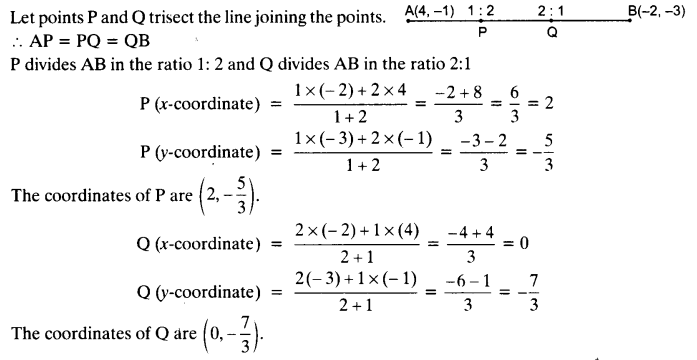Question 3.
To conduct Sports Day activities, in your rectangular shaped school ground ABCD, lines have been drawn with chalk powder at a distance of 1 m each. 100 flower pots have been placed at a distance of 1 m from each other along AD, as shown in given
figure below. Niharika runs $$\frac { 1 }{ 4 }$$ th the distance AD on the 2nd line and posts a green flag. Preet runs $$\frac { 1 }{ 5 }$$ th distance AD on the eighth line and posts a red flag. What is the distance between both the flags? If Rashmi has to post a blue flag exactly halfway between the line segment joining the two flags, where should she post her flag?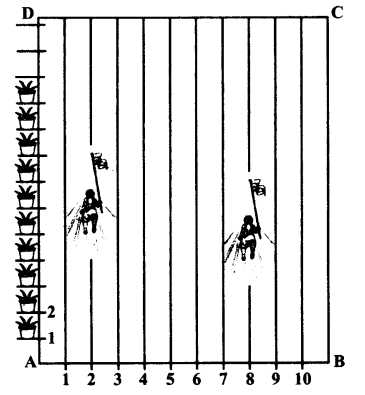Solution:
y-coordinate of green flag = $$\frac { 1 }{ 4 }$$ x 100 m = 25 m
Coordinates of green flag are P (2, 25)
y-coordinate of red flag = $$\frac { 1 }{ 5 }$$ x 100 = 20
Coordinates of red flag are Q (8, 20)
The distance between two points is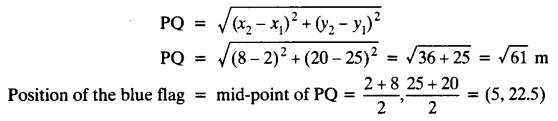The blue flag is in the 5th line, at a distance of 22.5 m.

Question 4.
Find the ratio in which the line segment joining the points (-3, 10) and (6, -8) is divided by (-1, 6).
Solution:
Let the required ratio be k : 1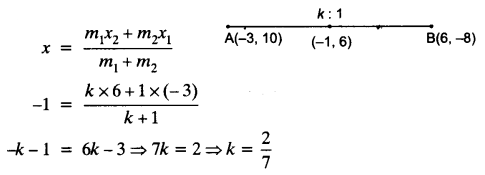Question 5.
Find the ratio in which line segment joining A (1, -5) and B (-4, 5) is divided by the x-axis. Also, find the coordinates of the point of division.
Solution: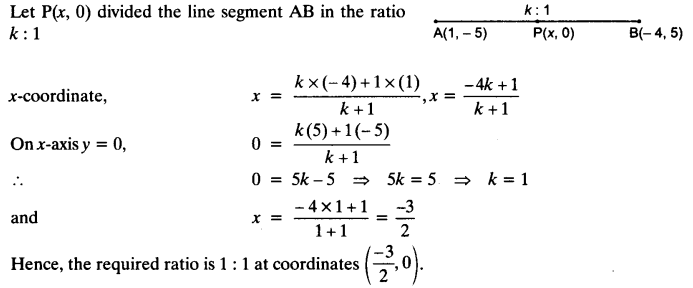Question 6.
If (1, 2), (4, y), (x, 6) and (3, 5) are the vertices of a parallelogram taken in order, find x and y.
Solution:Question 7.
Find the coordinates of a point A, where AB is the diameter of a circle whose centre is (2, -3) and B is (1, 4).
Solution: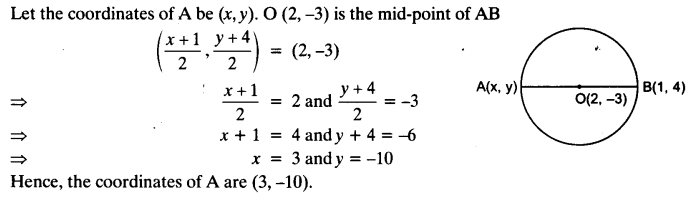Question 8.
If A and B are (-2, -2) and (2, -4), respectively, find the coordinates of P such that AP = $$\frac { 3 }{ 7 }$$ AB and P lies on the line segment AB.
Solution: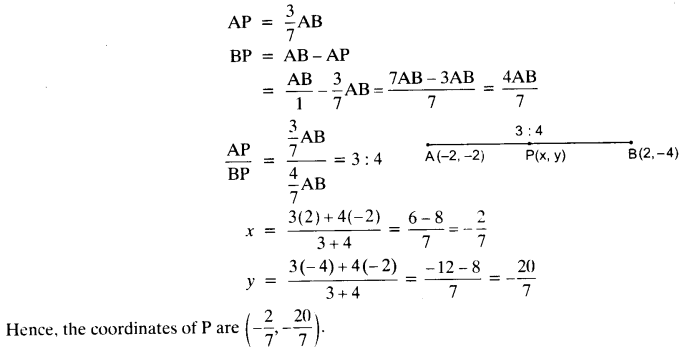Question 9.
Find the coordinates of the points which divide the line segment joining A (-2, 2) and B (2, 8) into four equal parts.
Solution:Question 10.
Find the area of a rhombus if its vertices are (3, 0), (4, 5), (-1, 4) and (-2, -1) taken in order.
[Hint: Area of a rhombus = $$\frac { 1 }{ 2 }$$ (product of its diagonals)]
Solution:We hope the NCERT Solutions for Class 10 Maths Chapter 7 Coordinate Geometry Ex 7.2, help you. If you have any query regarding NCERT Solutions for Class 10 Maths Chapter 7 Coordinate Geometry Ex 7.2, drop a comment below and we will get back to you at the earliest.